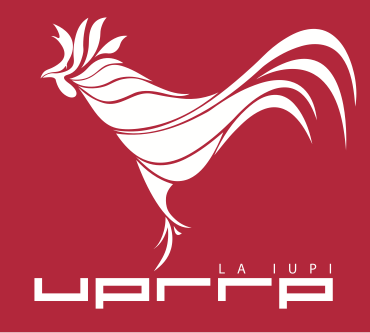## Seminar

A new class of almost automorphic functions and an application to monotone evolution equations,   Gaston M. N’Guérékata,   Morgan State University,  Fri, 11 September, 2009,   2:30 p.m. C-212

### Abstract

We will present the (new) class of (Stepanov-like) almost automorphic (Sp-a.e.) functions with value in a Banach space X. This class contains the classical almost automorphic functions in Bochner's sense. The results obtained are used to prove the existence and uniqueness of a weak Sp-a.a. solution to the parabolic equation u'(t)+A(t)u(t)=f(t) in a reflexive Banach space, assuming some properties of monotonicity and coercitivity of the operators A(t) and Sp'-almost automorphy of the forced term f. The result extends a well-known result in the case of almost periodicity. We will give an application to the heat equation and raise some open problems.